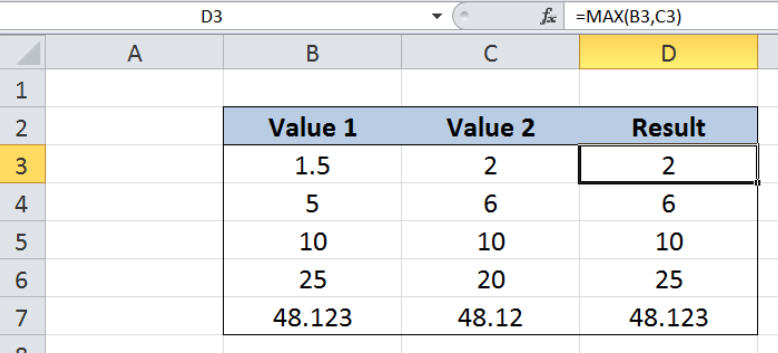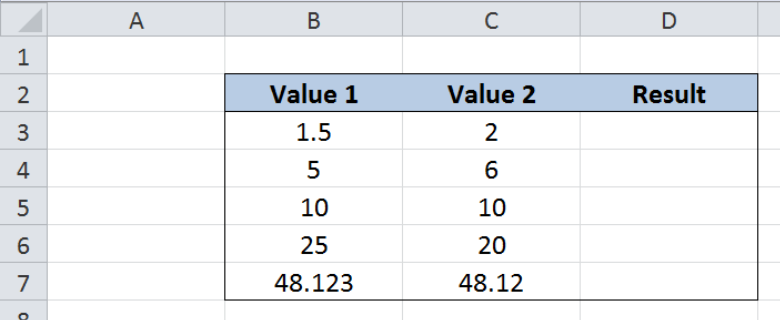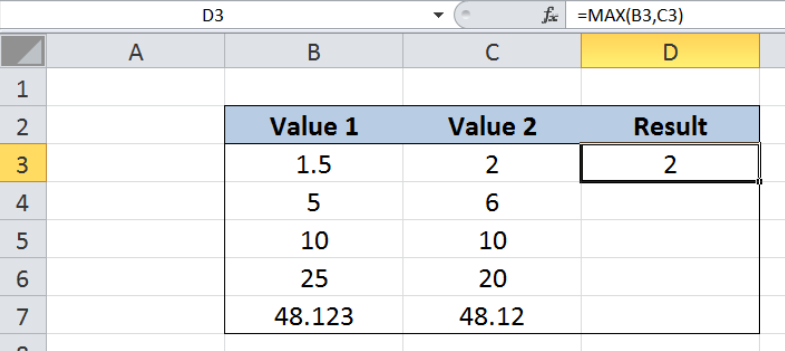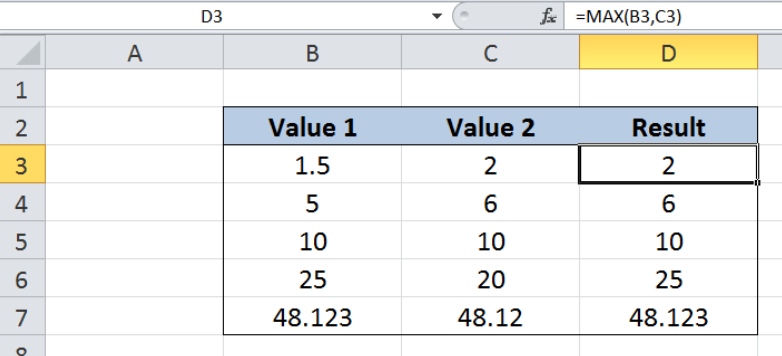Get instant live expert help with Excel or Google Sheets“My Excelchat expert helped me in less than 20 minutes, saving me what would have been 5 hours of work!”

#### Post your problem and you’ll get Expert help in seconds.

Your message must be at least 40 characters
Our professional Expert are available now. Your privacy is guaranteed.

# Larger of two valuesRead time: 20 minutes

While working with Excel, we are able to compare two or more values and determine the largest value by using the MAX function.  This step by step tutorial will assist all levels of Excel users in determining the larger of two values.Figure 1. Final result: Larger of two values

## Syntax of MAX Function

MAX function returns the largest value in a data set

`=MAX(number1, [number2], ...)`

• number1, number2, … – the numbers for which we want to find the largest value; only number1 is required; succeeding numbers are optional
• The arguments could be numbers, array or reference to cells containing numbers

## Setting up our Data

Our data consists of three columns : Value 1 (column B), Value 2 (column C) and Result (column D).  We want to compare the two values and determine the larger value. The results will be recorded in column D.Figure 2. Sample data  for larger of two values

## Larger of two values using MAX

In determining the larger of two values using MAX function, let us follow these steps:

Step 1.  Select cell D3

Step 2.  Enter the formula: `=MAX(B3,C3)`

Step 3.  Press ENTER

Step 4:  Copy the formula in cell D3 to cells D4:D7 by clicking the “+” icon at the bottom-right corner of cell D3 and dragging it down

As a result, the value in D3 is 2, because 2 is larger than 1.5.Figure 3.  Using MAX function to determine larger of two values

Below table shows the result in cells D3:D7, where MAX function returns the larger of the two values in every row.Figure 4.  Output: Larger of two values

## Larger of two values using IF

There is an alternative solution to determine the larger of two values by using the IF function.   Our formula in cell D3 becomes:

`=IF(B3>=C3,B3,C3)`

The IF function presents more uses than the MAX function, because we can customize the criteria and the values that will be returned by the formula.  However, in determining the larger of two values, the MAX function is shorter and simpler to use.

Most of the time, the problem you will need to solve will be more complex than a simple application of a formula or function. If you want to save hours of research and frustration, try our live Excelchat service! Our Excel Experts are available 24/7 to answer any Excel question you may have. We guarantee a connection within 30 seconds and a customized solution within 20 minutes.

### Did this post not answer your question? Get a solution from connecting with the expert.Another blog reader asked this question today on Excelchat:
Solution exampleshello i need help creating a formula, if a value does not exsits put 0, if a value exist do nothing
Solved by M. F. in 9 minsCompute the weighted average of students score from a spreadsheet using different weight for each category of marks
Solved by G. E. in 20 minshi there, im making a spread sheet to track progress in a game and would like to create a drop log sheet, i was hoping to use the if function to detect weather a item is logged on a sheet then send the sheet number to a list if so
Solved by K. F. in 60 minsI have a schedule I'm doing with formulas to add workdays (across rows) to a start date column. I would like to write the equation like this: =ifError((workday(b2,1) = cell above, [value of cell above + 1] Help me form this correctly to get the (value of target cell) = (1 more than the value from the cell above) IF the target cell value started equal to the cell above.
Solved by M. J. in 20 minsI need a formula that will subtract the sum of one cell if the sum of another cell is greater than zero, and have it applied to cells across a document that is 30,000 entries and only in certain spots
Solved by F. J. in 20 mins## Subscribe to Excelchat.coAnother blog reader asked this question today on Excelchat: